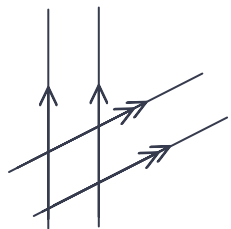Parallel and Perpendicular Lines

## Parallel and Perpendicular Lines

Parallel lines are always the same distance apart: they never meet. On drawings, parallel lines are shown with arrows. If there is more than one pair of parallel lines on a drawing, then the arrows are doubled up for the second pair.Perpendicular lines are shown with a square for the angle, rather than a curve.## Example 1

On a graph, are the lines x=0 and x=1 parallel or perpendicular?

x=0 and x=1 never meet, so the lines are parallel.